Successfully reported this slideshow.
We use your LinkedIn profile and activity data to personalize ads and to show you more relevant ads. You can change your ad preferences anytime.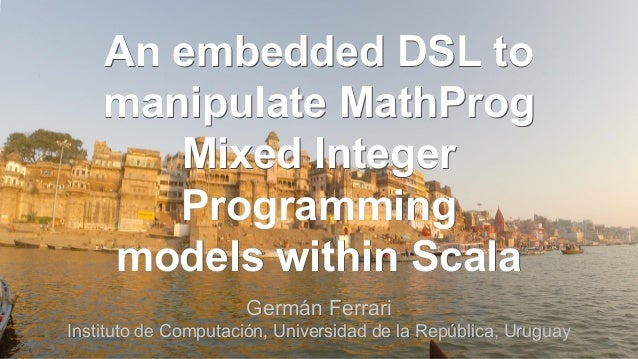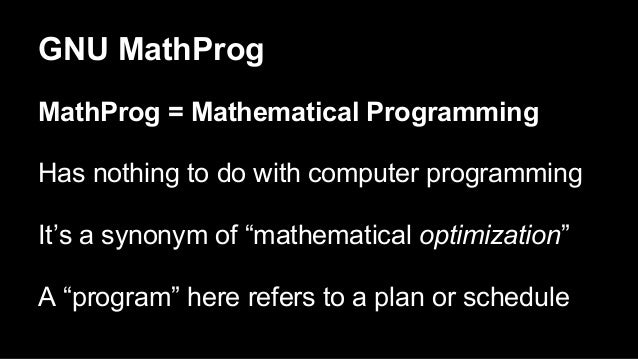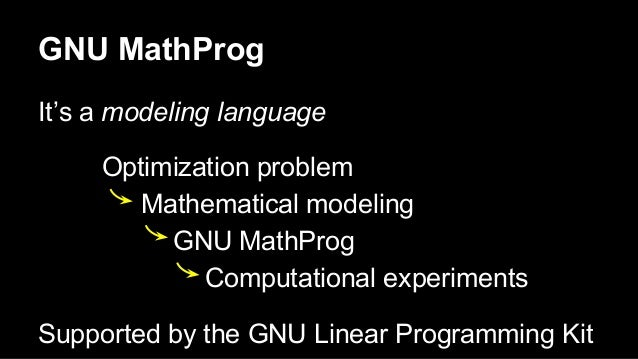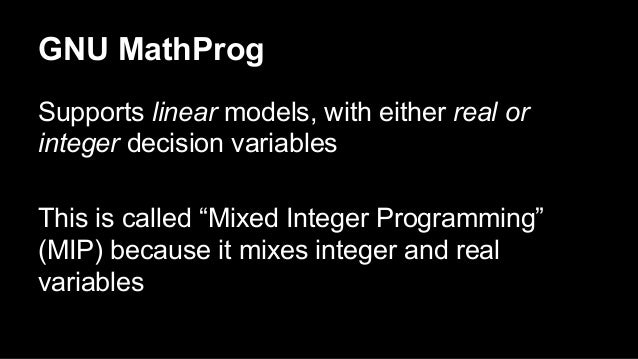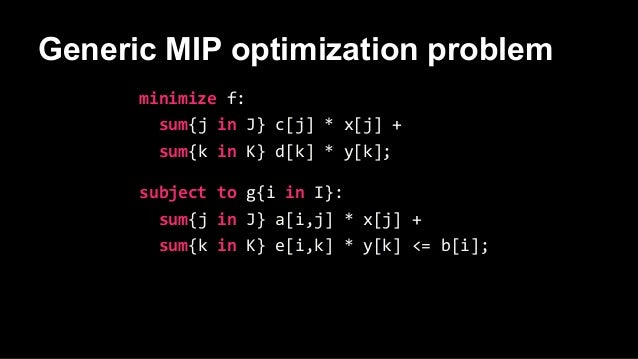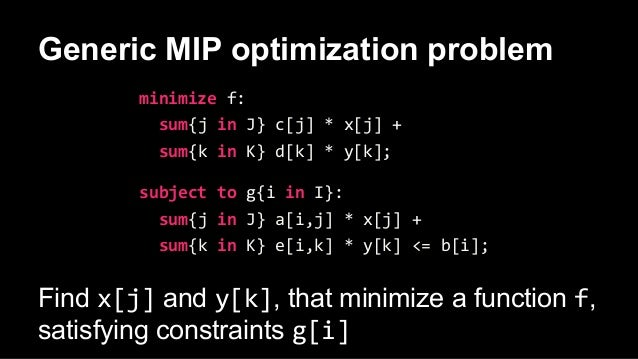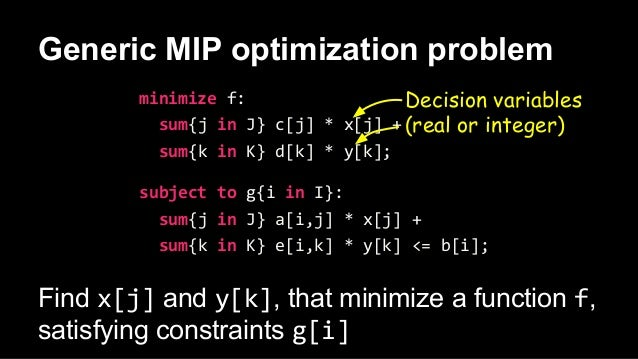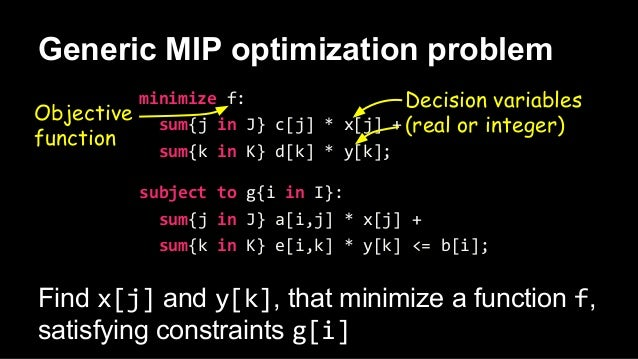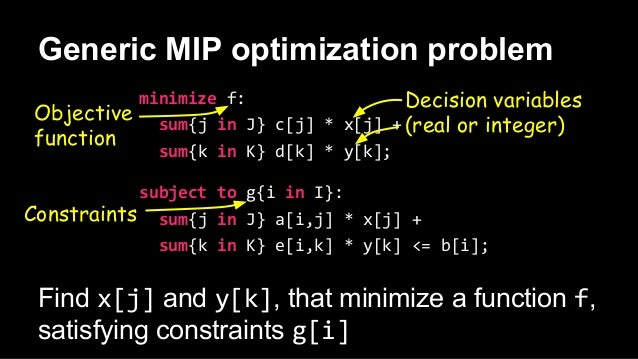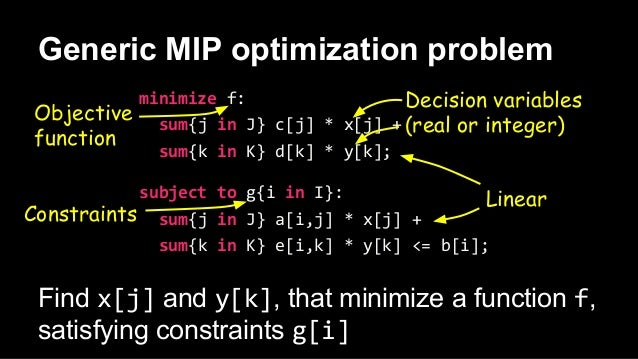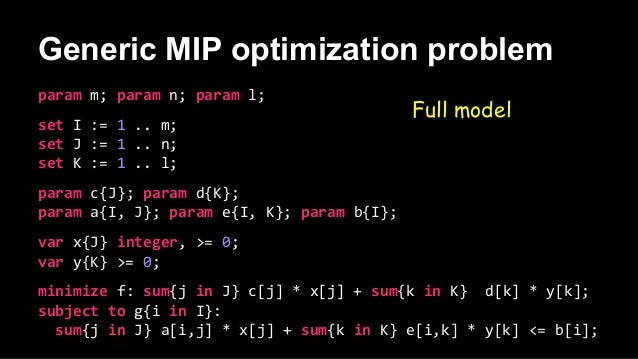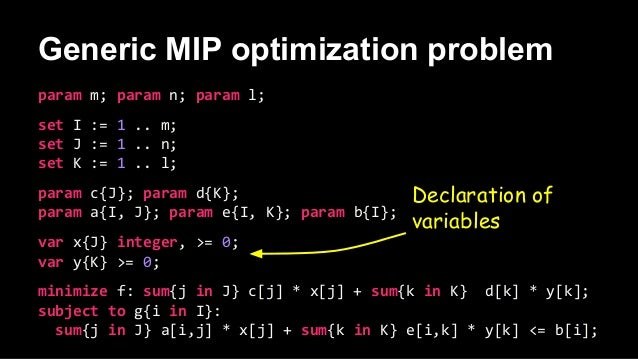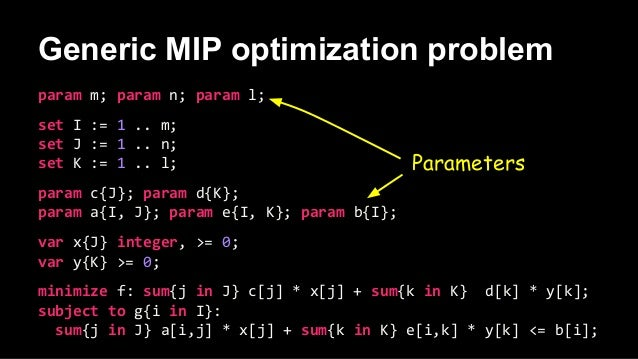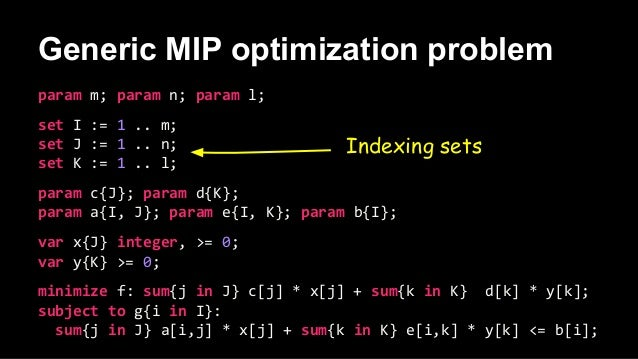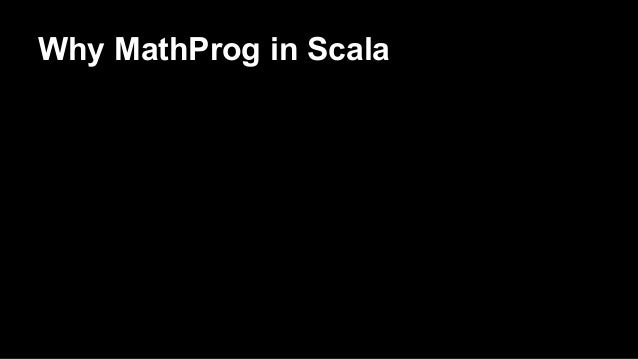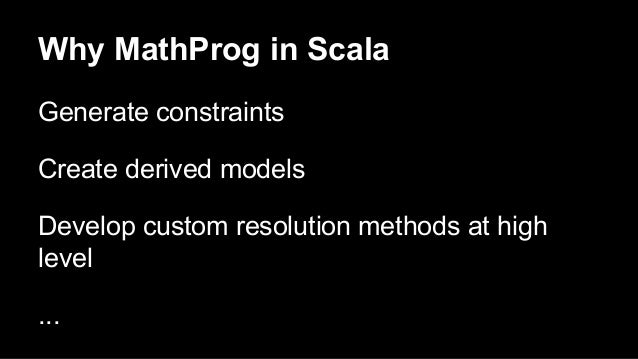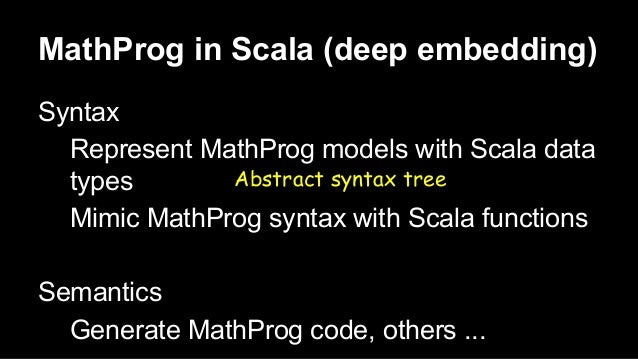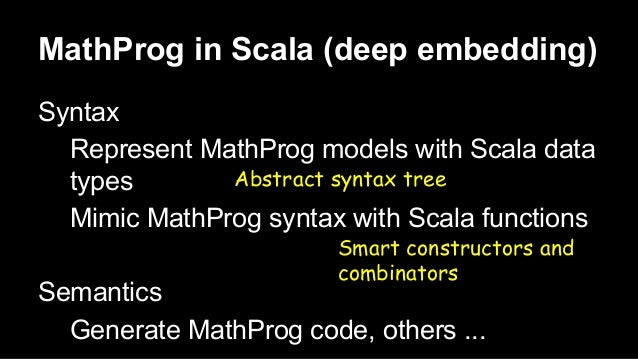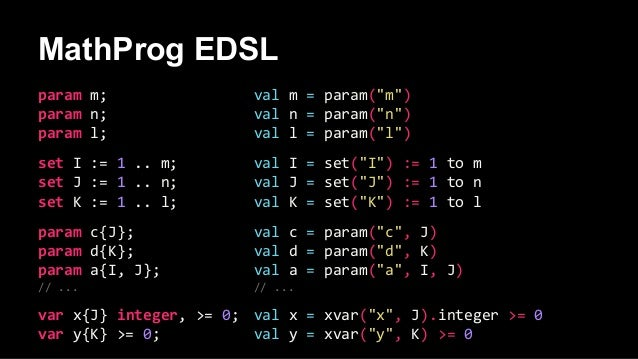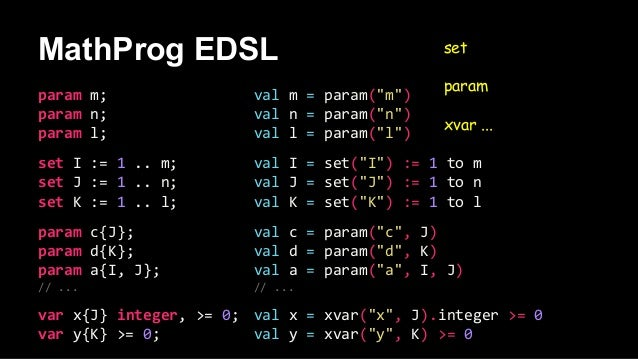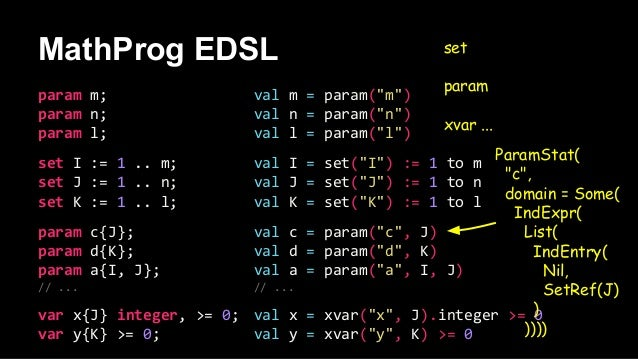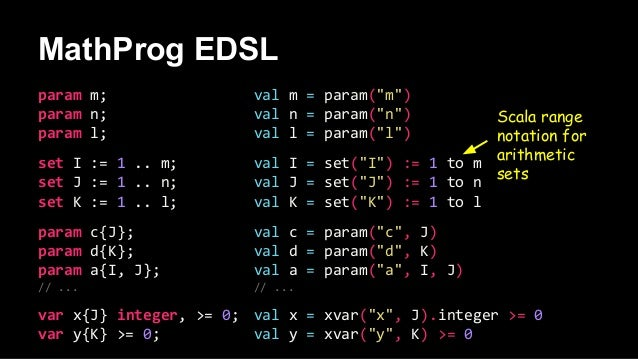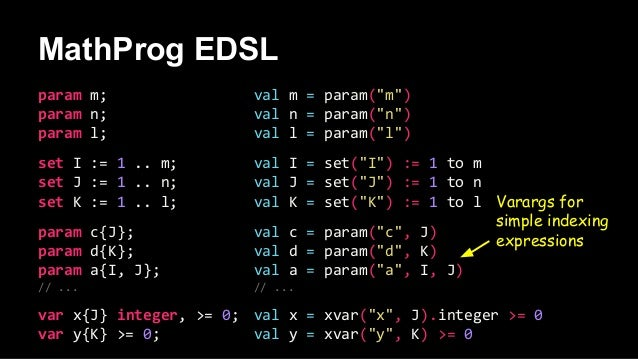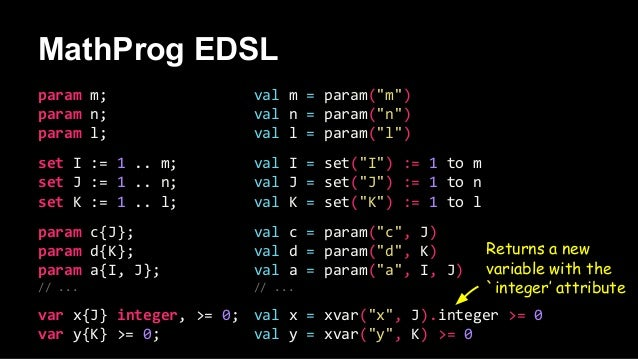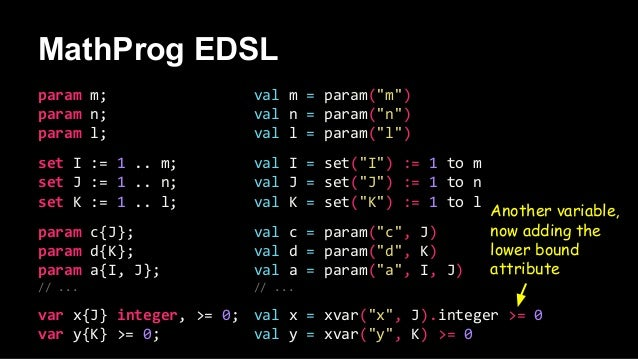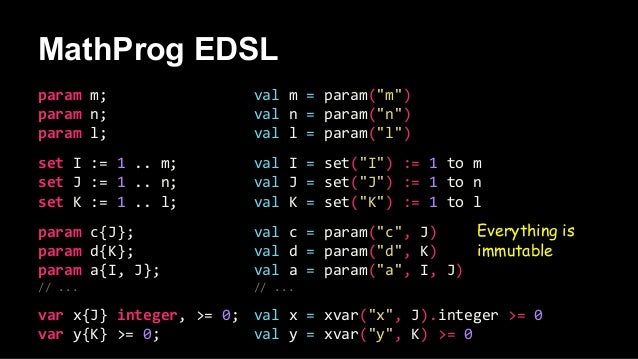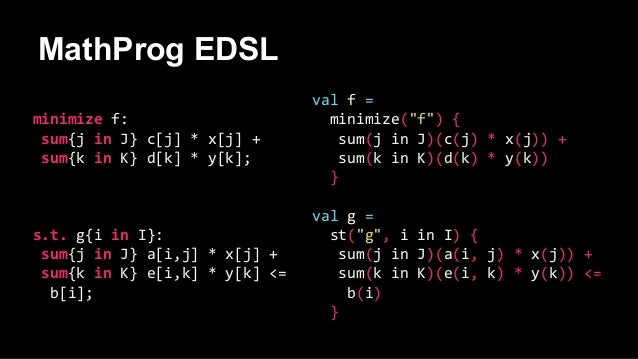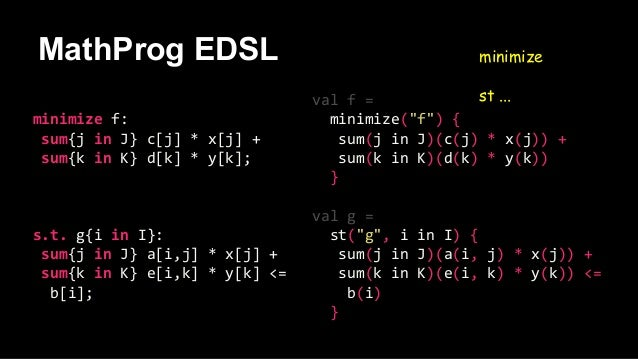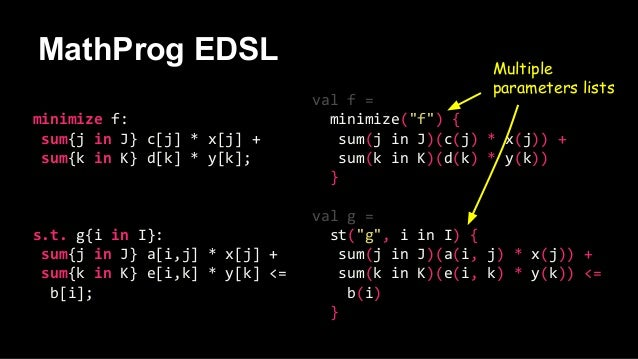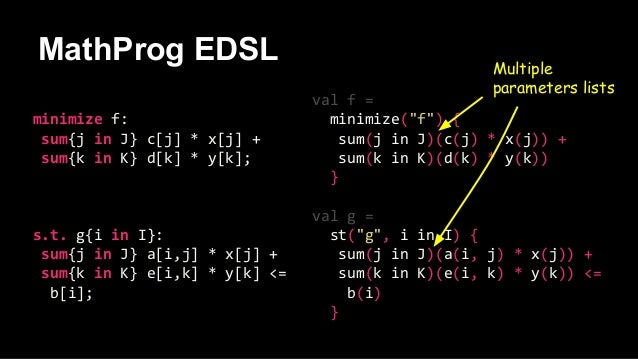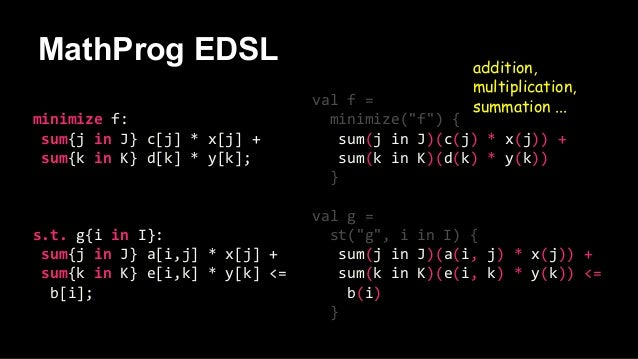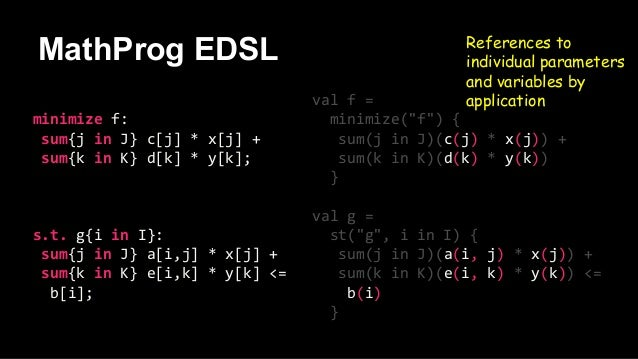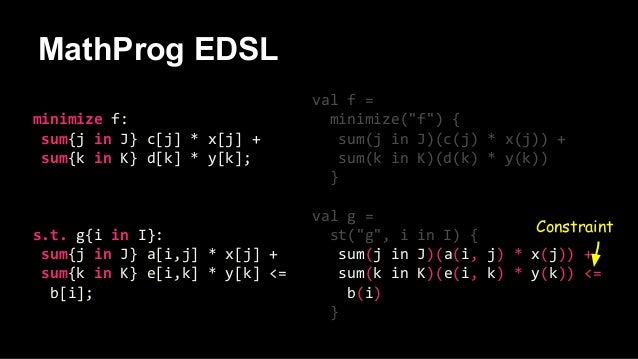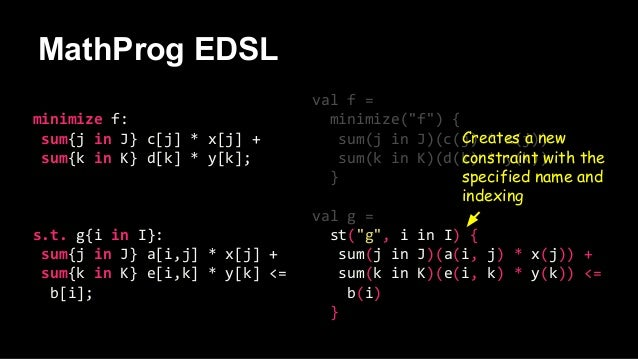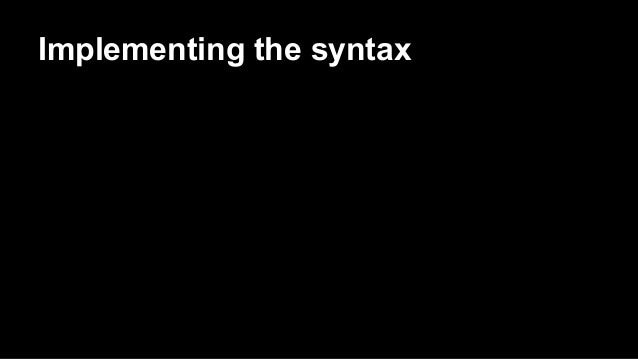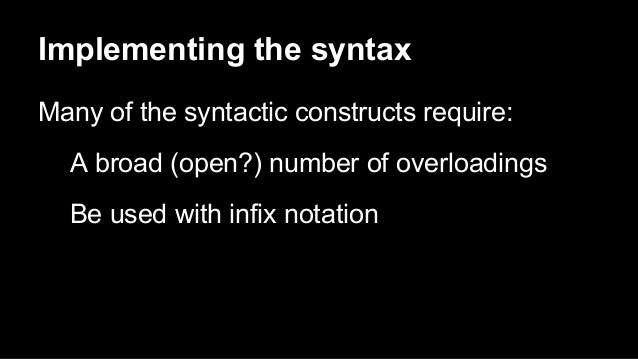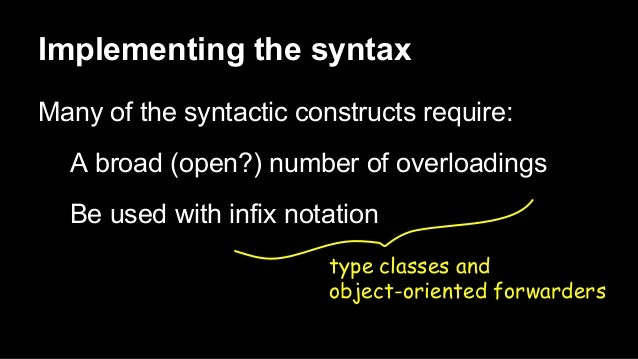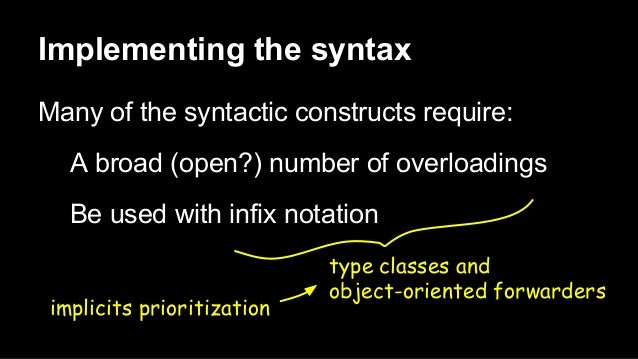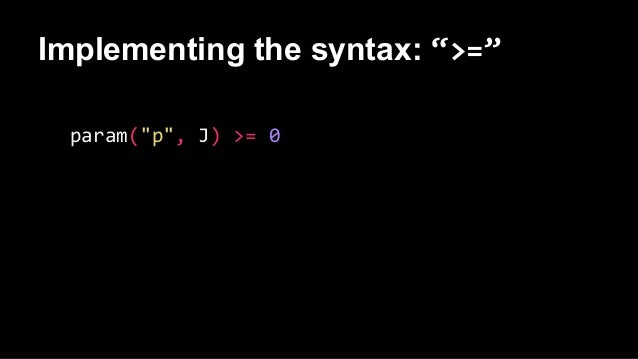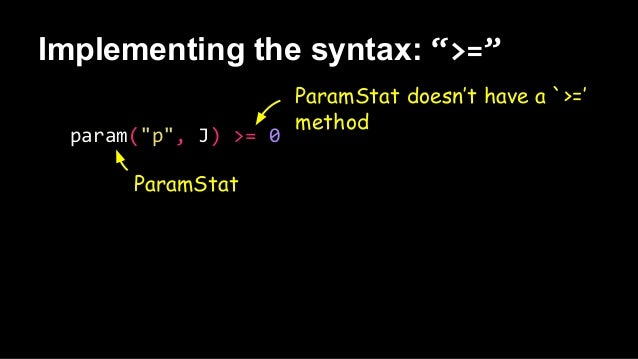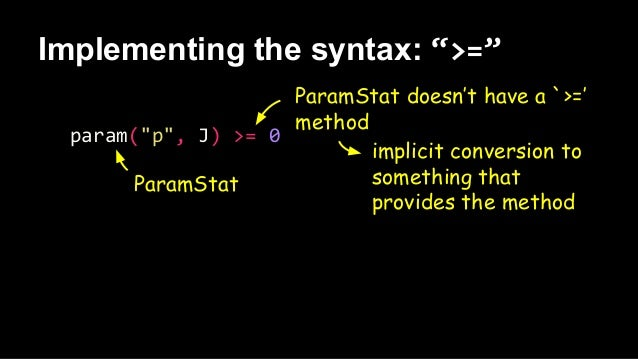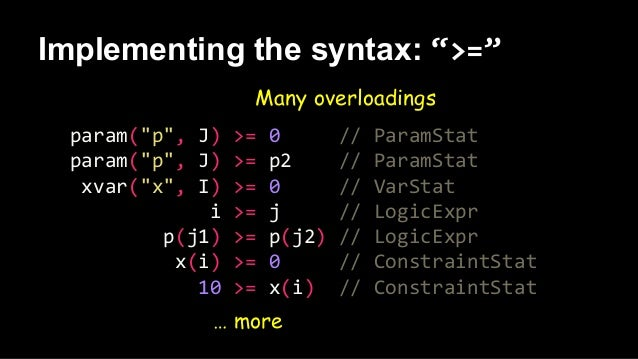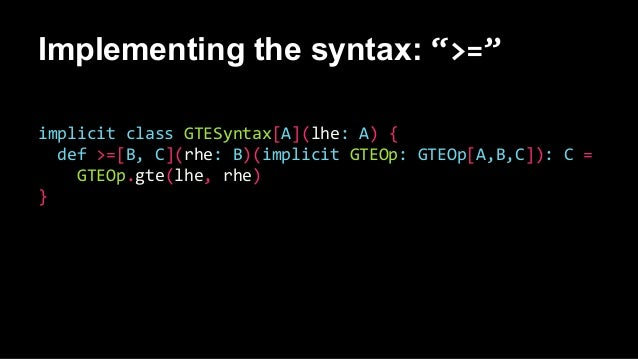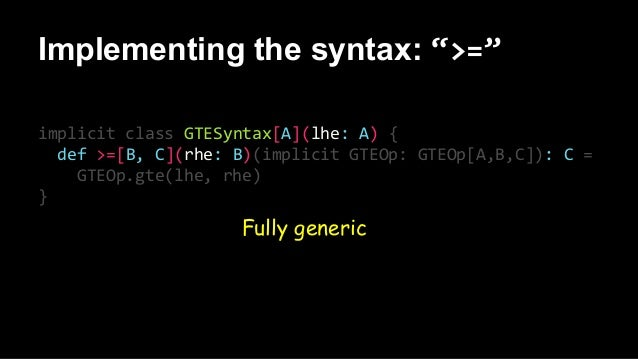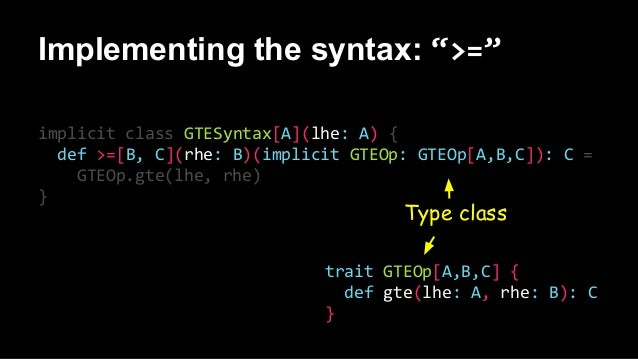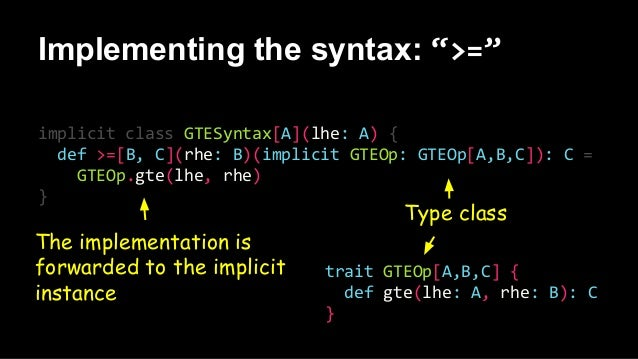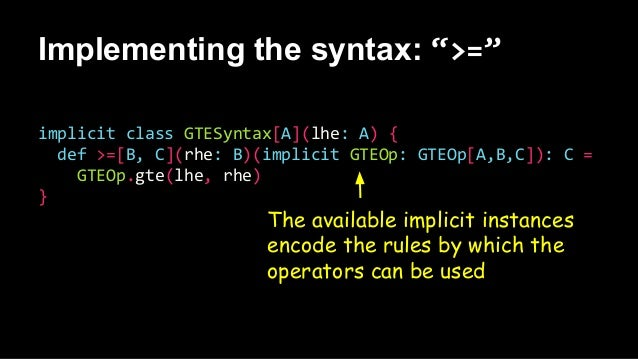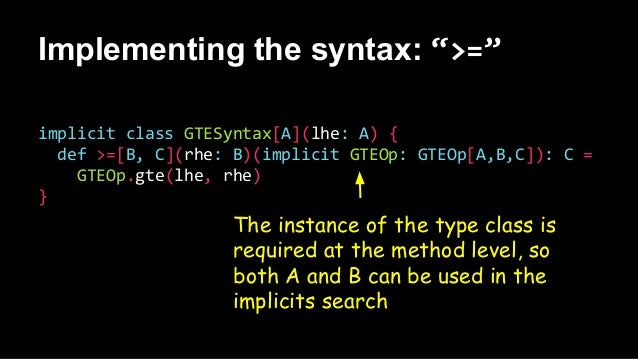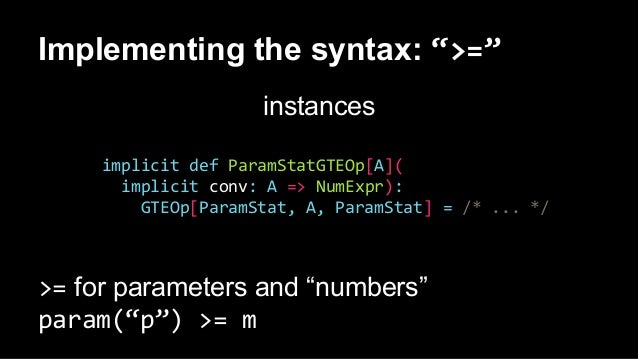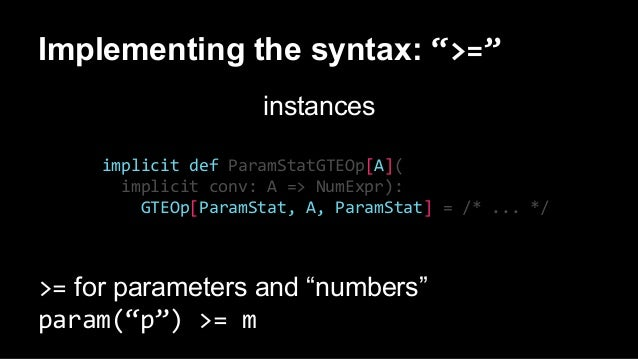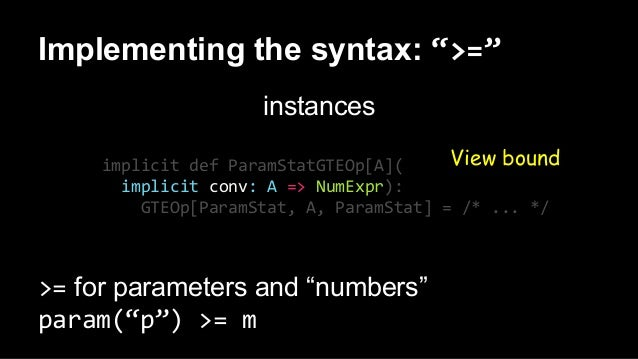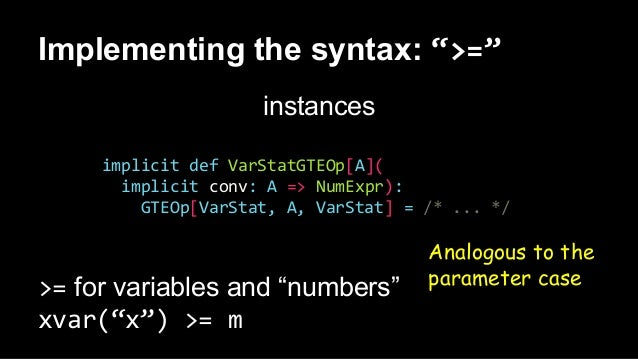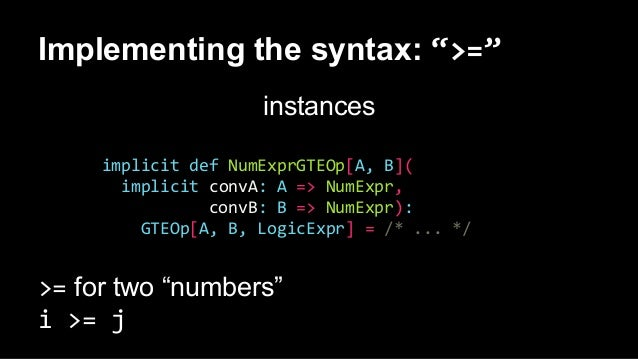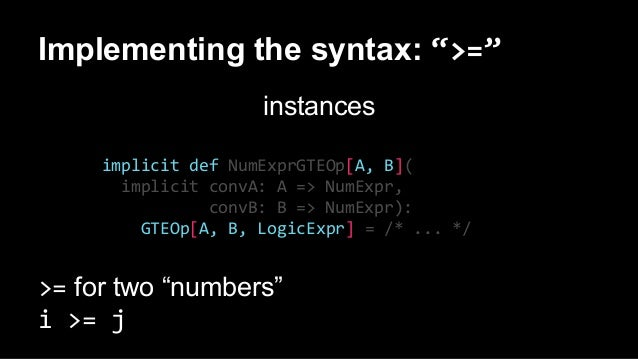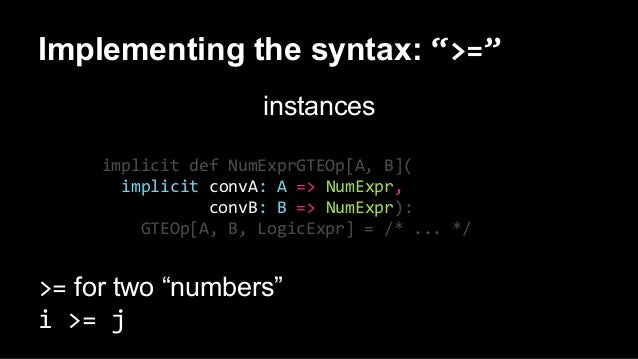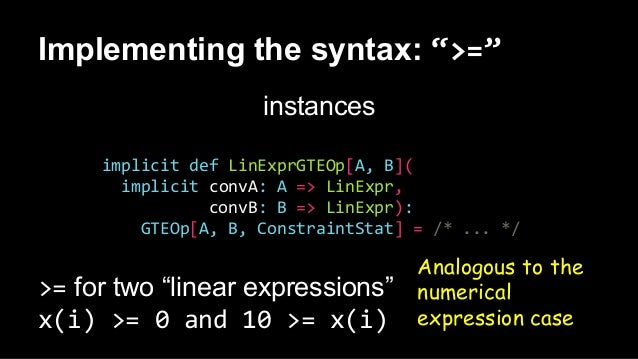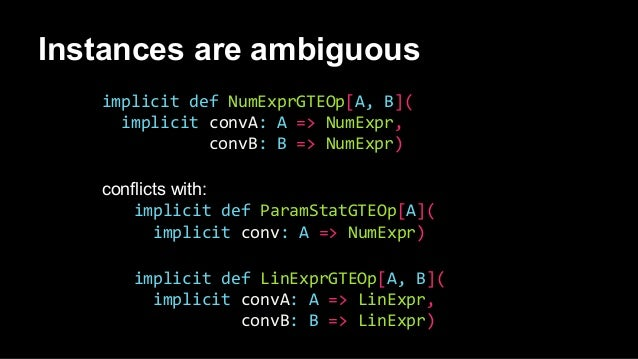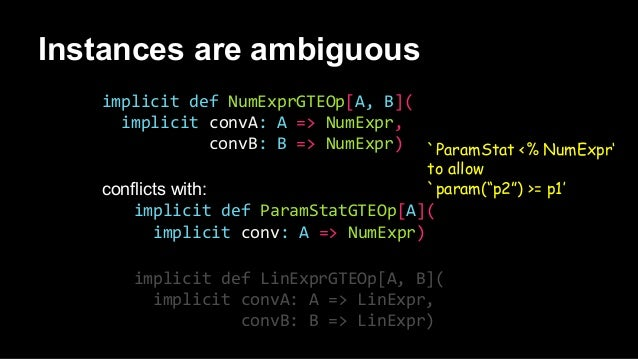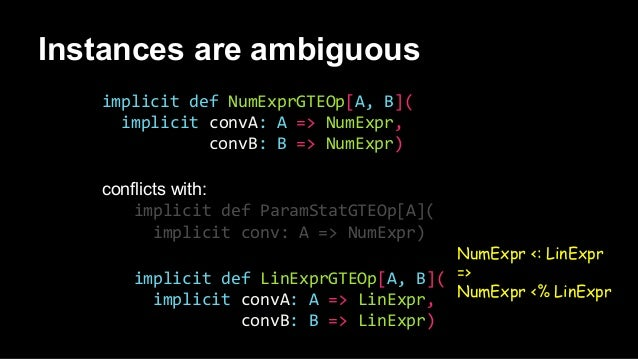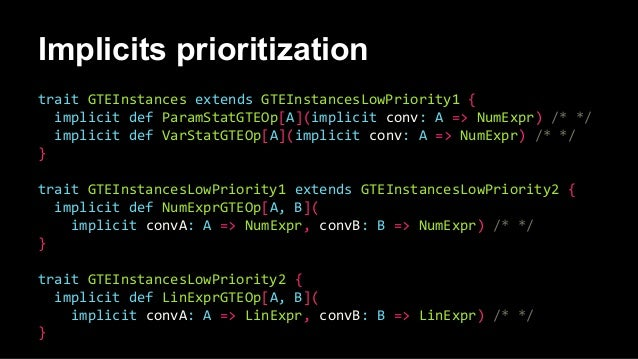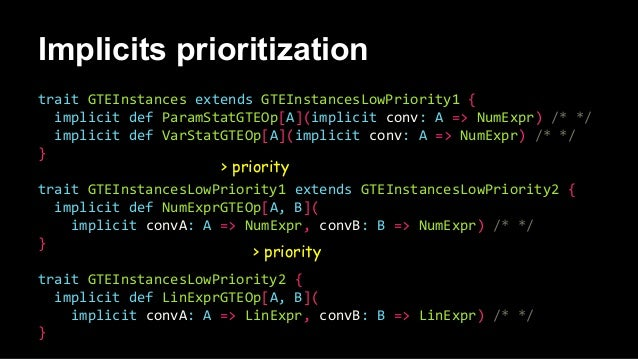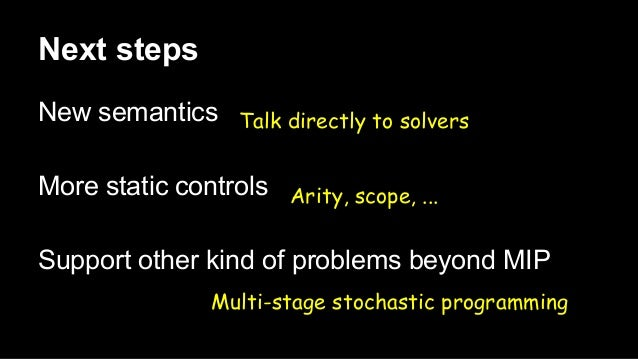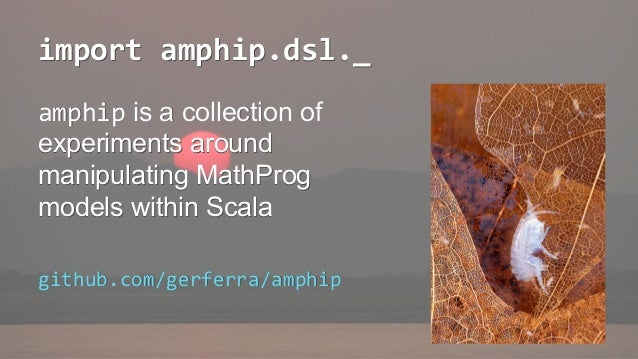Upcoming SlideShare
Loading in …5
×

# An embedded DSL to manipulate MathProg Mixed Integer Programming models within Scala

Slides for my talk at Scala By The Bay 2015 http://scalabythebay2015.sched.org/event/8cbbc52523ac9e5a905361e00d357099

GNU Mathprog is an algebraic modeling language for describing mathematical programming models. The language is a subset of AMPL, the most popular language used for describing such models. One advantage of this languages is the similarity of its syntax with the mathematical notation used to describe optimization problems. In this talk we describe the use of Scala in the construction of an embedded DSL to work with Mathprog models. At construction time, the DSL works as an alternative way to build the AST representing the model, taking advantage of the Scala compiler to check the validity of most expressions. The AST can be created also by parsing Mathprog model files. Once an AST is obtained, the DSL allows to manipulate and transform the AST to extract data or create new models. We show how the use of type classes and implicit conversion prioritization gives us a systematic way to define operators, valid combinations of parameters and result types, and conversions between expressions.

https://github.com/gerferra/amphip

• Full Name
Comment goes here.

Are you sure you want to Yes No
Your message goes here• Be the first to comment

### An embedded DSL to manipulate MathProg Mixed Integer Programming models within Scala

1. 1. Germán Ferrari Instituto de Computación, Universidad de la República, Uruguay An embedded DSL to manipulate MathProg Mixed Integer Programming models within Scala An embedded DSL to manipulate MathProg Mixed Integer Programming models within Scala Germán Ferrari Instituto de Computación, Universidad de la República, Uruguay
2. 2. GNU MathProg MathProg = Mathematical Programming Has nothing to do with computer programming It’s a synonym of “mathematical optimization” A “program” here refers to a plan or schedule
3. 3. GNU MathProg It’s a modeling language Optimization problem Mathematical modeling GNU MathProg Computational experiments Supported by the GNU Linear Programming Kit
4. 4. GNU MathProg Supports linear models, with either real or integer decision variables This is called “Mixed Integer Programming” (MIP) because it mixes integer and real variables
5. 5. Generic MIP optimization problem minimize f: sum{j in J} c[j] * x[j] + sum{k in K} d[k] * y[k]; subject to g{i in I}: sum{j in J} a[i,j] * x[j] + sum{k in K} e[i,k] * y[k] <= b[i];
6. 6. Generic MIP optimization problem Find x[j] and y[k], that minimize a function f, satisfying constraints g[i] minimize f: sum{j in J} c[j] * x[j] + sum{k in K} d[k] * y[k]; subject to g{i in I}: sum{j in J} a[i,j] * x[j] + sum{k in K} e[i,k] * y[k] <= b[i];
7. 7. Generic MIP optimization problem Find x[j] and y[k], that minimize a function f, satisfying constraints g[i] minimize f: sum{j in J} c[j] * x[j] + sum{k in K} d[k] * y[k]; subject to g{i in I}: sum{j in J} a[i,j] * x[j] + sum{k in K} e[i,k] * y[k] <= b[i]; Decision variables (real or integer)
8. 8. Generic MIP optimization problem Find x[j] and y[k], that minimize a function f, satisfying constraints g[i] minimize f: sum{j in J} c[j] * x[j] + sum{k in K} d[k] * y[k]; subject to g{i in I}: sum{j in J} a[i,j] * x[j] + sum{k in K} e[i,k] * y[k] <= b[i]; Decision variables (real or integer) Objective function
9. 9. Generic MIP optimization problem Find x[j] and y[k], that minimize a function f, satisfying constraints g[i] minimize f: sum{j in J} c[j] * x[j] + sum{k in K} d[k] * y[k]; subject to g{i in I}: sum{j in J} a[i,j] * x[j] + sum{k in K} e[i,k] * y[k] <= b[i]; Decision variables (real or integer) Objective function Constraints
10. 10. Generic MIP optimization problem Find x[j] and y[k], that minimize a function f, satisfying constraints g[i] minimize f: sum{j in J} c[j] * x[j] + sum{k in K} d[k] * y[k]; subject to g{i in I}: sum{j in J} a[i,j] * x[j] + sum{k in K} e[i,k] * y[k] <= b[i]; Decision variables (real or integer) Objective function Constraints Linear
11. 11. Generic MIP optimization problem Full model param m; param n; param l; set I := 1 .. m; set J := 1 .. n; set K := 1 .. l; param c{J}; param d{K}; param a{I, J}; param e{I, K}; param b{I}; var x{J} integer, >= 0; var y{K} >= 0; minimize f: sum{j in J} c[j] * x[j] + sum{k in K} d[k] * y[k]; subject to g{i in I}: sum{j in J} a[i,j] * x[j] + sum{k in K} e[i,k] * y[k] <= b[i];
12. 12. param m; param n; param l; set I := 1 .. m; set J := 1 .. n; set K := 1 .. l; param c{J}; param d{K}; param a{I, J}; param e{I, K}; param b{I}; var x{J} integer, >= 0; var y{K} >= 0; minimize f: sum{j in J} c[j] * x[j] + sum{k in K} d[k] * y[k]; subject to g{i in I}: sum{j in J} a[i,j] * x[j] + sum{k in K} e[i,k] * y[k] <= b[i]; Generic MIP optimization problem Declaration of variables
13. 13. param m; param n; param l; set I := 1 .. m; set J := 1 .. n; set K := 1 .. l; param c{J}; param d{K}; param a{I, J}; param e{I, K}; param b{I}; var x{J} integer, >= 0; var y{K} >= 0; minimize f: sum{j in J} c[j] * x[j] + sum{k in K} d[k] * y[k]; subject to g{i in I}: sum{j in J} a[i,j] * x[j] + sum{k in K} e[i,k] * y[k] <= b[i]; Generic MIP optimization problem Parameters
14. 14. Generic MIP optimization problem param m; param n; param l; set I := 1 .. m; set J := 1 .. n; set K := 1 .. l; param c{J}; param d{K}; param a{I, J}; param e{I, K}; param b{I}; var x{J} integer, >= 0; var y{K} >= 0; minimize f: sum{j in J} c[j] * x[j] + sum{k in K} d[k] * y[k]; subject to g{i in I}: sum{j in J} a[i,j] * x[j] + sum{k in K} e[i,k] * y[k] <= b[i]; Indexing sets
15. 15. Why MathProg in Scala
16. 16. Why MathProg in Scala Generate constraints Create derived models Develop custom resolution methods at high level ...
17. 17. MathProg in Scala (deep embedding) Syntax Represent MathProg models with Scala data types Mimic MathProg syntax with Scala functions Semantics Generate MathProg code, others ...
18. 18. MathProg in Scala (deep embedding) Syntax Represent MathProg models with Scala data types Mimic MathProg syntax with Scala functions Semantics Generate MathProg code, others ... Abstract syntax tree
19. 19. MathProg in Scala (deep embedding) Syntax Represent MathProg models with Scala data types Mimic MathProg syntax with Scala functions Semantics Generate MathProg code, others ... Smart constructors and combinators Abstract syntax tree
20. 20. MathProg EDSL param m; param n; param l; set I := 1 .. m; set J := 1 .. n; set K := 1 .. l; param c{J}; param d{K}; param a{I, J}; // ... var x{J} integer, >= 0; var y{K} >= 0; val m = param("m") val n = param("n") val l = param("l") val I = set("I") := 1 to m val J = set("J") := 1 to n val K = set("K") := 1 to l val c = param("c", J) val d = param("d", K) val a = param("a", I, J) // ... val x = xvar("x", J).integer >= 0 val y = xvar("y", K) >= 0
21. 21. MathProg EDSL param m; param n; param l; set I := 1 .. m; set J := 1 .. n; set K := 1 .. l; param c{J}; param d{K}; param a{I, J}; // ... var x{J} integer, >= 0; var y{K} >= 0; val m = param("m") val n = param("n") val l = param("l") val I = set("I") := 1 to m val J = set("J") := 1 to n val K = set("K") := 1 to l val c = param("c", J) val d = param("d", K) val a = param("a", I, J) // ... val x = xvar("x", J).integer >= 0 val y = xvar("y", K) >= 0 set param xvar ...
22. 22. MathProg EDSL param m; param n; param l; set I := 1 .. m; set J := 1 .. n; set K := 1 .. l; param c{J}; param d{K}; param a{I, J}; // ... var x{J} integer, >= 0; var y{K} >= 0; val m = param("m") val n = param("n") val l = param("l") val I = set("I") := 1 to m val J = set("J") := 1 to n val K = set("K") := 1 to l val c = param("c", J) val d = param("d", K) val a = param("a", I, J) // ... val x = xvar("x", J).integer >= 0 val y = xvar("y", K) >= 0 set param xvar ... ParamStat( "c", domain = Some( IndExpr( List( IndEntry( Nil, SetRef(J) ) ))))
23. 23. val m = param("m") val n = param("n") val l = param("l") val I = set("I") := 1 to m val J = set("J") := 1 to n val K = set("K") := 1 to l val c = param("c", J) val d = param("d", K) val a = param("a", I, J) // ... val x = xvar("x", J).integer >= 0 val y = xvar("y", K) >= 0 MathProg EDSL Scala range notation for arithmetic sets param m; param n; param l; set I := 1 .. m; set J := 1 .. n; set K := 1 .. l; param c{J}; param d{K}; param a{I, J}; // ... var x{J} integer, >= 0; var y{K} >= 0;
24. 24. val m = param("m") val n = param("n") val l = param("l") val I = set("I") := 1 to m val J = set("J") := 1 to n val K = set("K") := 1 to l val c = param("c", J) val d = param("d", K) val a = param("a", I, J) // ... val x = xvar("x", J).integer >= 0 val y = xvar("y", K) >= 0 param m; param n; param l; set I := 1 .. m; set J := 1 .. n; set K := 1 .. l; param c{J}; param d{K}; param a{I, J}; // ... var x{J} integer, >= 0; var y{K} >= 0; MathProg EDSL Varargs for simple indexing expressions
25. 25. val m = param("m") val n = param("n") val l = param("l") val I = set("I") := 1 to m val J = set("J") := 1 to n val K = set("K") := 1 to l val c = param("c", J) val d = param("d", K) val a = param("a", I, J) // ... val x = xvar("x", J).integer >= 0 val y = xvar("y", K) >= 0 param m; param n; param l; set I := 1 .. m; set J := 1 .. n; set K := 1 .. l; param c{J}; param d{K}; param a{I, J}; // ... var x{J} integer, >= 0; var y{K} >= 0; MathProg EDSL Returns a new variable with the `integer’ attribute
26. 26. val m = param("m") val n = param("n") val l = param("l") val I = set("I") := 1 to m val J = set("J") := 1 to n val K = set("K") := 1 to l val c = param("c", J) val d = param("d", K) val a = param("a", I, J) // ... val x = xvar("x", J).integer >= 0 val y = xvar("y", K) >= 0 MathProg EDSL Another variable, now adding the lower bound attribute param m; param n; param l; set I := 1 .. m; set J := 1 .. n; set K := 1 .. l; param c{J}; param d{K}; param a{I, J}; // ... var x{J} integer, >= 0; var y{K} >= 0;
27. 27. val m = param("m") val n = param("n") val l = param("l") val I = set("I") := 1 to m val J = set("J") := 1 to n val K = set("K") := 1 to l val c = param("c", J) val d = param("d", K) val a = param("a", I, J) // ... val x = xvar("x", J).integer >= 0 val y = xvar("y", K) >= 0 param m; param n; param l; set I := 1 .. m; set J := 1 .. n; set K := 1 .. l; param c{J}; param d{K}; param a{I, J}; // ... var x{J} integer, >= 0; var y{K} >= 0; MathProg EDSL Everything is immutable
28. 28. MathProg EDSL minimize f: sum{j in J} c[j] * x[j] + sum{k in K} d[k] * y[k]; s.t. g{i in I}: sum{j in J} a[i,j] * x[j] + sum{k in K} e[i,k] * y[k] <= b[i]; val f = minimize("f") { sum(j in J)(c(j) * x(j)) + sum(k in K)(d(k) * y(k)) } val g = st("g", i in I) { sum(j in J)(a(i, j) * x(j)) + sum(k in K)(e(i, k) * y(k)) <= b(i) }
29. 29. MathProg EDSL minimize st ... minimize f: sum{j in J} c[j] * x[j] + sum{k in K} d[k] * y[k]; s.t. g{i in I}: sum{j in J} a[i,j] * x[j] + sum{k in K} e[i,k] * y[k] <= b[i]; val f = minimize("f") { sum(j in J)(c(j) * x(j)) + sum(k in K)(d(k) * y(k)) } val g = st("g", i in I) { sum(j in J)(a(i, j) * x(j)) + sum(k in K)(e(i, k) * y(k)) <= b(i) }
30. 30. minimize f: sum{j in J} c[j] * x[j] + sum{k in K} d[k] * y[k]; s.t. g{i in I}: sum{j in J} a[i,j] * x[j] + sum{k in K} e[i,k] * y[k] <= b[i]; val f = minimize("f") { sum(j in J)(c(j) * x(j)) + sum(k in K)(d(k) * y(k)) } val g = st("g", i in I) { sum(j in J)(a(i, j) * x(j)) + sum(k in K)(e(i, k) * y(k)) <= b(i) } MathProg EDSL Multiple parameters lists
31. 31. minimize f: sum{j in J} c[j] * x[j] + sum{k in K} d[k] * y[k]; s.t. g{i in I}: sum{j in J} a[i,j] * x[j] + sum{k in K} e[i,k] * y[k] <= b[i]; val f = minimize("f") { sum(j in J)(c(j) * x(j)) + sum(k in K)(d(k) * y(k)) } val g = st("g", i in I) { sum(j in J)(a(i, j) * x(j)) + sum(k in K)(e(i, k) * y(k)) <= b(i) } MathProg EDSL Multiple parameters lists
32. 32. minimize f: sum{j in J} c[j] * x[j] + sum{k in K} d[k] * y[k]; s.t. g{i in I}: sum{j in J} a[i,j] * x[j] + sum{k in K} e[i,k] * y[k] <= b[i]; val f = minimize("f") { sum(j in J)(c(j) * x(j)) + sum(k in K)(d(k) * y(k)) } val g = st("g", i in I) { sum(j in J)(a(i, j) * x(j)) + sum(k in K)(e(i, k) * y(k)) <= b(i) } MathProg EDSL addition, multiplication, summation ...
33. 33. minimize f: sum{j in J} c[j] * x[j] + sum{k in K} d[k] * y[k]; s.t. g{i in I}: sum{j in J} a[i,j] * x[j] + sum{k in K} e[i,k] * y[k] <= b[i]; val f = minimize("f") { sum(j in J)(c(j) * x(j)) + sum(k in K)(d(k) * y(k)) } val g = st("g", i in I) { sum(j in J)(a(i, j) * x(j)) + sum(k in K)(e(i, k) * y(k)) <= b(i) } MathProg EDSL References to individual parameters and variables by application
34. 34. minimize f: sum{j in J} c[j] * x[j] + sum{k in K} d[k] * y[k]; s.t. g{i in I}: sum{j in J} a[i,j] * x[j] + sum{k in K} e[i,k] * y[k] <= b[i]; val f = minimize("f") { sum(j in J)(c(j) * x(j)) + sum(k in K)(d(k) * y(k)) } val g = st("g", i in I) { sum(j in J)(a(i, j) * x(j)) + sum(k in K)(e(i, k) * y(k)) <= b(i) } MathProg EDSL Constraint
35. 35. minimize f: sum{j in J} c[j] * x[j] + sum{k in K} d[k] * y[k]; s.t. g{i in I}: sum{j in J} a[i,j] * x[j] + sum{k in K} e[i,k] * y[k] <= b[i]; val f = minimize("f") { sum(j in J)(c(j) * x(j)) + sum(k in K)(d(k) * y(k)) } val g = st("g", i in I) { sum(j in J)(a(i, j) * x(j)) + sum(k in K)(e(i, k) * y(k)) <= b(i) } MathProg EDSL Creates a new constraint with the specified name and indexing
36. 36. Implementing the syntax
37. 37. Implementing the syntax Many of the syntactic constructs require: A broad (open?) number of overloadings Be used with infix notation
38. 38. Many of the syntactic constructs require: A broad (open?) number of overloadings Be used with infix notation Implementing the syntax type classes and object-oriented forwarders
39. 39. Many of the syntactic constructs require: A broad (open?) number of overloadings Be used with infix notation Implementing the syntax type classes and object-oriented forwarders implicits prioritization
40. 40. Implementing the syntax: “>=” param("p", J) >= 0
41. 41. Implementing the syntax: “>=” param("p", J) >= 0 ParamStat
42. 42. Implementing the syntax: “>=” param("p", J) >= 0 ParamStat ParamStat doesn’t have a `>=’ method
43. 43. Implementing the syntax: “>=” param("p", J) >= 0 implicit conversion to something that provides the method ParamStat doesn’t have a `>=’ method ParamStat
44. 44. Implementing the syntax: “>=” param("p", J) >= 0 // ParamStat param("p", J) >= p2 // ParamStat xvar("x", I) >= 0 // VarStat i >= j // LogicExpr p(j1) >= p(j2) // LogicExpr x(i) >= 0 // ConstraintStat 10 >= x(i) // ConstraintStat Many overloadings … more
45. 45. Implementing the syntax: “>=” implicit class GTESyntax[A](lhe: A) { def >=[B, C](rhe: B)(implicit GTEOp: GTEOp[A,B,C]): C = GTEOp.gte(lhe, rhe) }
46. 46. Implementing the syntax: “>=” implicit class GTESyntax[A](lhe: A) { def >=[B, C](rhe: B)(implicit GTEOp: GTEOp[A,B,C]): C = GTEOp.gte(lhe, rhe) } Fully generic
47. 47. Implementing the syntax: “>=” implicit class GTESyntax[A](lhe: A) { def >=[B, C](rhe: B)(implicit GTEOp: GTEOp[A,B,C]): C = GTEOp.gte(lhe, rhe) } trait GTEOp[A,B,C] { def gte(lhe: A, rhe: B): C } Type class
48. 48. Implementing the syntax: “>=” implicit class GTESyntax[A](lhe: A) { def >=[B, C](rhe: B)(implicit GTEOp: GTEOp[A,B,C]): C = GTEOp.gte(lhe, rhe) } trait GTEOp[A,B,C] { def gte(lhe: A, rhe: B): C } Type class The implementation is forwarded to the implicit instance
49. 49. Implementing the syntax: “>=” implicit class GTESyntax[A](lhe: A) { def >=[B, C](rhe: B)(implicit GTEOp: GTEOp[A,B,C]): C = GTEOp.gte(lhe, rhe) } The available implicit instances encode the rules by which the operators can be used
50. 50. Implementing the syntax: “>=” implicit class GTESyntax[A](lhe: A) { def >=[B, C](rhe: B)(implicit GTEOp: GTEOp[A,B,C]): C = GTEOp.gte(lhe, rhe) } The instance of the type class is required at the method level, so both A and B can be used in the implicits search
51. 51. Implementing the syntax: “>=” instances implicit def ParamStatGTEOp[A]( implicit conv: A => NumExpr): GTEOp[ParamStat, A, ParamStat] = /* ... */ >= for parameters and “numbers” param(“p”) >= m
52. 52. Implementing the syntax: “>=” instances implicit def ParamStatGTEOp[A]( implicit conv: A => NumExpr): GTEOp[ParamStat, A, ParamStat] = /* ... */ >= for parameters and “numbers” param(“p”) >= m
53. 53. Implementing the syntax: “>=” instances implicit def ParamStatGTEOp[A]( implicit conv: A => NumExpr): GTEOp[ParamStat, A, ParamStat] = /* ... */ >= for parameters and “numbers” param(“p”) >= m View bound
54. 54. Implementing the syntax: “>=” instances implicit def VarStatGTEOp[A]( implicit conv: A => NumExpr): GTEOp[VarStat, A, VarStat] = /* ... */ >= for variables and “numbers” xvar(“x”) >= m Analogous to the parameter case
55. 55. Implementing the syntax: “>=” instances implicit def NumExprGTEOp[A, B]( implicit convA: A => NumExpr, convB: B => NumExpr): GTEOp[A, B, LogicExpr] = /* ... */ >= for two “numbers” i >= j
56. 56. Implementing the syntax: “>=” instances implicit def NumExprGTEOp[A, B]( implicit convA: A => NumExpr, convB: B => NumExpr): GTEOp[A, B, LogicExpr] = /* ... */ >= for two “numbers” i >= j
57. 57. Implementing the syntax: “>=” instances implicit def NumExprGTEOp[A, B]( implicit convA: A => NumExpr, convB: B => NumExpr): GTEOp[A, B, LogicExpr] = /* ... */ >= for two “numbers” i >= j
58. 58. Implementing the syntax: “>=” instances implicit def LinExprGTEOp[A, B]( implicit convA: A => LinExpr, convB: B => LinExpr): GTEOp[A, B, ConstraintStat] = /* ... */ >= for two “linear expressions” x(i) >= 0 and 10 >= x(i) Analogous to the numerical expression case
59. 59. implicit def NumExprGTEOp[A, B]( implicit convA: A => NumExpr, convB: B => NumExpr) conflicts with: implicit def ParamStatGTEOp[A]( implicit conv: A => NumExpr) implicit def LinExprGTEOp[A, B]( implicit convA: A => LinExpr, convB: B => LinExpr) Instances are ambiguous
60. 60. implicit def NumExprGTEOp[A, B]( implicit convA: A => NumExpr, convB: B => NumExpr) conflicts with: implicit def ParamStatGTEOp[A]( implicit conv: A => NumExpr) implicit def LinExprGTEOp[A, B]( implicit convA: A => LinExpr, convB: B => LinExpr) Instances are ambiguous `ParamStat <% NumExpr‘ to allow `param(“p2”) >= p1’
61. 61. Instances are ambiguous implicit def NumExprGTEOp[A, B]( implicit convA: A => NumExpr, convB: B => NumExpr) conflicts with: implicit def ParamStatGTEOp[A]( implicit conv: A => NumExpr) implicit def LinExprGTEOp[A, B]( implicit convA: A => LinExpr, convB: B => LinExpr) NumExpr <: LinExpr => NumExpr <% LinExpr
62. 62. Implicits prioritization trait GTEInstances extends GTEInstancesLowPriority1 { implicit def ParamStatGTEOp[A](implicit conv: A => NumExpr) /* */ implicit def VarStatGTEOp[A](implicit conv: A => NumExpr) /* */ } trait GTEInstancesLowPriority1 extends GTEInstancesLowPriority2 { implicit def NumExprGTEOp[A, B]( implicit convA: A => NumExpr, convB: B => NumExpr) /* */ } trait GTEInstancesLowPriority2 { implicit def LinExprGTEOp[A, B]( implicit convA: A => LinExpr, convB: B => LinExpr) /* */ }
63. 63. Implicits prioritization trait GTEInstances extends GTEInstancesLowPriority1 { implicit def ParamStatGTEOp[A](implicit conv: A => NumExpr) /* */ implicit def VarStatGTEOp[A](implicit conv: A => NumExpr) /* */ } trait GTEInstancesLowPriority1 extends GTEInstancesLowPriority2 { implicit def NumExprGTEOp[A, B]( implicit convA: A => NumExpr, convB: B => NumExpr) /* */ } trait GTEInstancesLowPriority2 { implicit def LinExprGTEOp[A, B]( implicit convA: A => LinExpr, convB: B => LinExpr) /* */ } > priority > priority
64. 64. Next steps New semantics More static controls Support other kind of problems beyond MIP Talk directly to solvers Arity, scope, ... Multi-stage stochastic programming
65. 65. import amphip.dsl._import amphip.dsl._ amphip is a collection of experiments around manipulating MathProg models within Scala github.com/gerferra/amphip amphip is a collection of experiments around manipulating MathProg models within Scala github.com/gerferra/amphip
66. 66. FIN github.com/gerferra/amphip